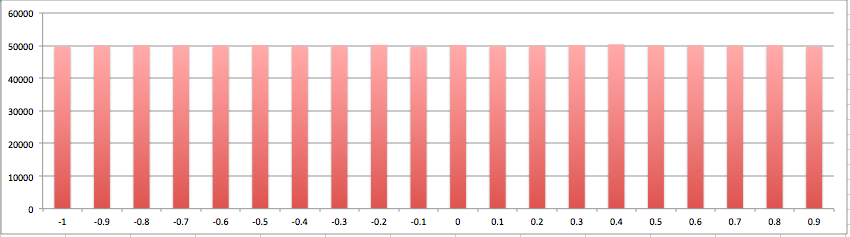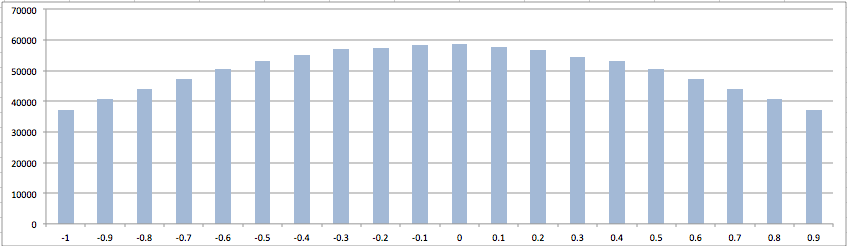Home » Java » Core Java » java.util.Random in Java 8

### About Biju Kunjummen# java.util.Random in Java 8

One of the neat features of java.util.Random class in Java 8 is that it has been retrofitted to now return a random Stream of numbers.

For eg, to generate an infinite stream of random doubles between 0(inclusive) and 1(exclusive):

```Random random = new Random();
DoubleStream doubleStream = random.doubles();```

or to generate an infinite stream of integers between 0(inclusive) and 100(exclusive):

```Random random = new Random();
IntStream intStream = random.ints(0, 100);```

So what can this infinite random stream be used for, I will demonstrate a few scenarios, do keep in mind though that since this is an infinite stream, any terminal operations has to be done once the stream has been made limited in some way, otherwise the operation will not terminate!

For eg. to get a stream of 10 random integers and print them:

`intStream.limit(10).forEach(System.out::println);`

Or to generate a list of 100 random integers :

```List<Integer> randomBetween0And99 = intStream
.limit(100)
.boxed()
.collect(Collectors.toList());```

For gaussian pseudo-random values, there is no stream equivalent of random.doubles(), however it is easy to come up with one with the facility that Java 8 provides:

```Random random = new Random();
DoubleStream gaussianStream = Stream.generate(random::nextGaussian).mapToDouble(e -> e);```

Here, I am using the Stream.generate api call passing in a Supplier which generates the next gaussian with the already available method in Random class.

So now to do something a little more interesting with the stream of pseudo-random doubles and the stream of Gaussian pseudo-random doubles, what I want to do is to get the distribution of doubles for each of these two streams, the expectation is that the distribution when plotted should be uniformly distributed for pseudo-random doubles and should be normal distributed for Gaussian pseudo-random doubles.

In the following code, I am generating such a distribution for a million pseudo-random values, this uses a lot of facilities provided by the new Java 8 Streams API:

```Random random = new Random();
DoubleStream doubleStream = random.doubles(-1.0, 1.0);
LinkedHashMap<Range, Integer> rangeCountMap = doubleStream.limit(1000000)
.boxed()
.map(Ranges::of)
.collect(Ranges::emptyRangeCountMap, (m, e) -> m.put(e, m.get(e) + 1), Ranges::mergeRangeCountMaps);

rangeCountMap.forEach((k, v) -> System.out.println(k.from() + "\t" + v));```

and this code spits out data along these lines:

```-1	49730
-0.9	49931
-0.8	50057
-0.7	50060
-0.6	49963
-0.5	50159
-0.4	49921
-0.3	49962
-0.2	50231
-0.1	49658
0	50177
0.1	49861
0.2	49947
0.3	50157
0.4	50414
0.5	50006
0.6	50038
0.7	49962
0.8	50071
0.9	49695```

and similarly generating a distribution for a million Gaussian pseudo-random values:

```Random random = new Random();
DoubleStream gaussianStream = Stream.generate(random::nextGaussian).mapToDouble(e -> e);
LinkedHashMap<Range, Integer> gaussianRangeCountMap =
gaussianStream
.filter(e -> (e >= -1.0 && e < 1.0))
.limit(1000000)
.boxed()
.map(Ranges::of)
.collect(Ranges::emptyRangeCountMap, (m, e) -> m.put(e, m.get(e) + 1), Ranges::mergeRangeCountMaps);

gaussianRangeCountMap.forEach((k, v) -> System.out.println(k.from() + "\t" + v));```

Plotting the data gives the expected result:

For a pseudo-random Data:And for Gaussian Data:• Complete code is available in a gist here – https://gist.github.com/bijukunjummen/8129250

Reference: java.util.Random in Java 8 from our JCG partner Biju Kunjummen at the all and sundry blog.(0 rating, 0 votes)
You need to be a registered member to rate this.
Start the discussion Views Tweet it!
Do you want to know how to develop your skillset to become a Java Rockstar?
Subscribe to our newsletter to start Rocking right now!
To get you started we give you our best selling eBooks for FREE!
1. JPA Mini Book
2. JVM Troubleshooting Guide
3. JUnit Tutorial for Unit Testing
4. Java Annotations Tutorial
5. Java Interview Questions
6. Spring Interview Questions
7. Android UI Design
and many more ....
I agree to the Terms and Privacy Policy